# Telling the Time to 5 Minute Intervals

Telling the Time to 5 Minute Intervals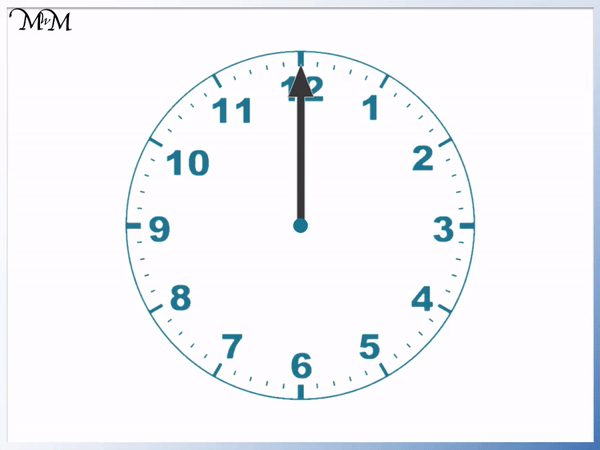• Each of the small marks on the edge of a clock face represents 1 minute.
• There are 5 minutes between each of the numbers that are written on the clock face.
• To see how many minutes past the hour each written number represents, we can multiply the number by 5.
• For example 2 × 5 = 10 and so, the 2 represents 10 minutes past.
• There are 60 minutes in a complete hour.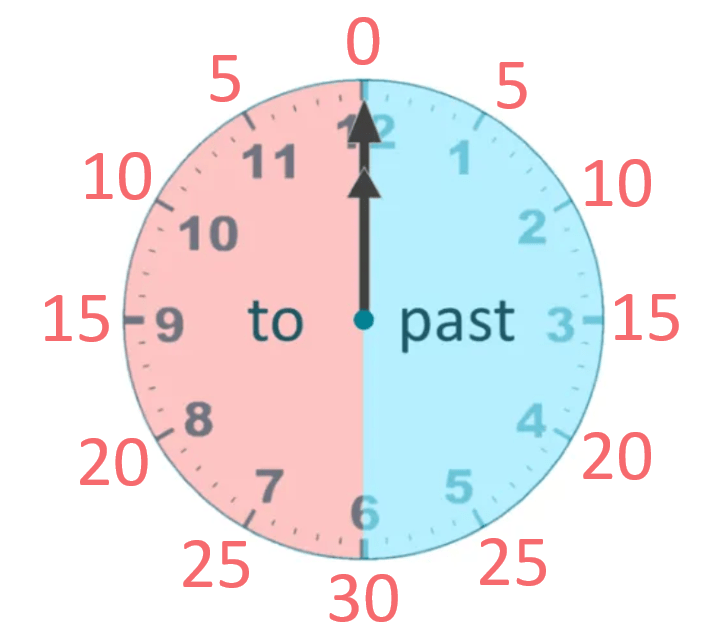• As well as representing minutes past the hour, the numbers on the clock can represent minuts to the hour.
• We can count backwards to see how many minutes to the hour each number represents.
• There are 5 minutes between each number written on the clock.
• The 11 represents 5 minutes to the hour.
• The 10 represents 10 minutes to the hour.
• We can count how many numbers each number is backwards from 12 and multiply this by 5 to see how many minutes to the hour it represents.
• We can see below that the minutes to and minutes past the hour are symmetrical.We can multiply each number on the clock by 5 to see how many minutes past the hour it represents.

The minutes to the hour are symmetrical with the minutes past the hour.• The longer minute hand is pointing at the 5.
• We multiply this 5 by 5 to see how many minutes past the hour it represents.
• 5 × 5 = 25, so we have 25 minutes past the hour.
• The shorter hour hand has gone past the 2 but not yet reached the 3.
• The time is 25 minutes past 2.# Telling the Time in 5 Minute Intervals

## 5 Minute Intervals Past the Hour

To tell the time in 5 minute intervals:

• Multiply the number that the minute hand is pointing at by 5.
• The result is the number of minutes past the hour.
• The hour is the last number on the clock that the hour hand has gone past.

The minutes on a clock are shown by the small lines on the outside edge of the clock face.

There are 5 minutes between each of the numbers that are written on the clock face.Each number written on the clock face represents 5 minutes past the hour.Here in an example of telling the time to the nearest 5 minute interval.We first look at the longer minute hand.

It is pointing at the 1.

We multiply the number 1 by 5 to see how many minutes past the hour it represents.

1 × 5 = 5 and so, the 1 on the clock represents 5 minutes past the hour.

If you count the number of minute marks from the top of the clock, there will be 5 by the time you get to the 1.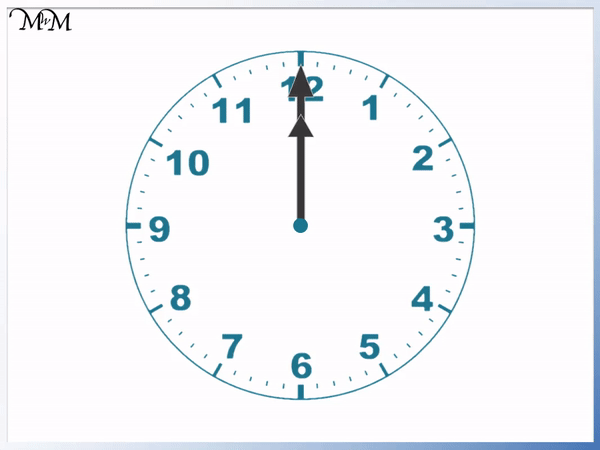The shorter hand is the hour hand and it has just gone past the 7.

This means that the time is 5 minutes past 7.

The hour hand tells us the hour and the minute hand tells us the minutes past this hour.

Here is another example of reading the minutes past the hour in 5 minute intervals.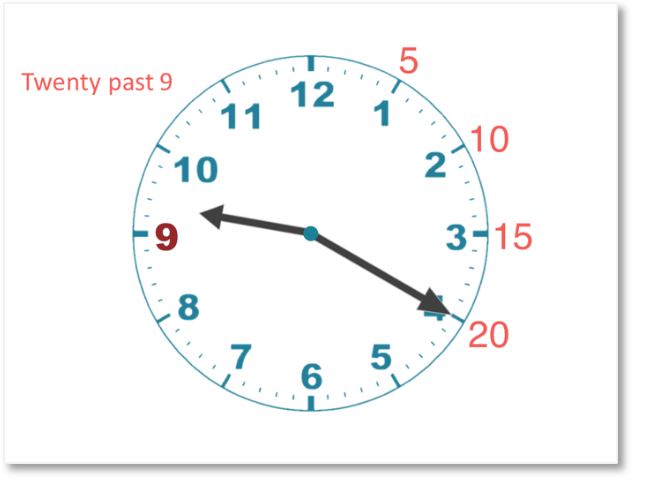The minute hand is pointing at the 4.

We multiply the 4 by 5 to see how many minutes past the hour this is.

4 × 5 = 20 and so, the 4 on the clock represents 20 minutes past the hour.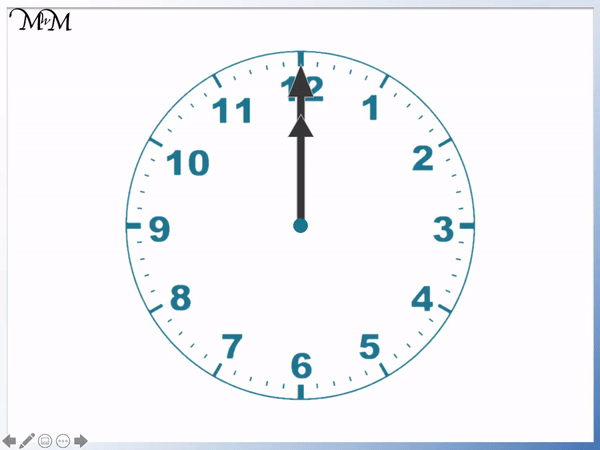The hour hand is in between the 9 and the 10. This means that the hour hand has past the 9 but not yet reached the 10.

The time is 20 minutes past 9.

Here is another example of telling the time to the nearest 5 minutes.

The minute hand is pointing at the 5.

We multiply 5 by 5 to see how many minutes past this represents.

5 × 5 = 25 and so, the 5 on the clock represents 25 minutes past the hour.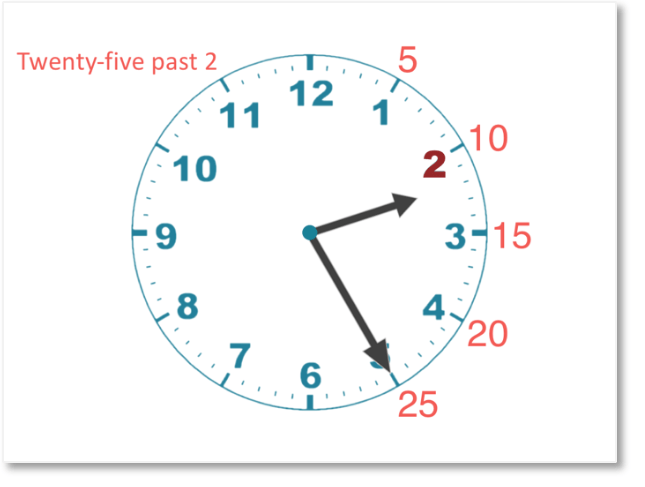The hour hand is in between 2 and 3. This means that the hour hand has gone past 2 but not yet reached 3.The time is 25 minutes past 2.

## Telling the Time in 5 Minute Intervals To the Hour

To tell the time in 5 minute intervals to the hour use the following steps:

• Look at the number on the clock that the minute hand is pointing to.
• Reading anticlockwise, count how many numbers there are until you get to this number.
• Multiply this amount by 5.
• This is the number of minutes to the hour.
• The hour is the number that the hour hand will point at next.

We look at minutes to the hour when the minutes hand has gone past the 6. This is when the minutes to the next hour is less than the minutes past the previous hour.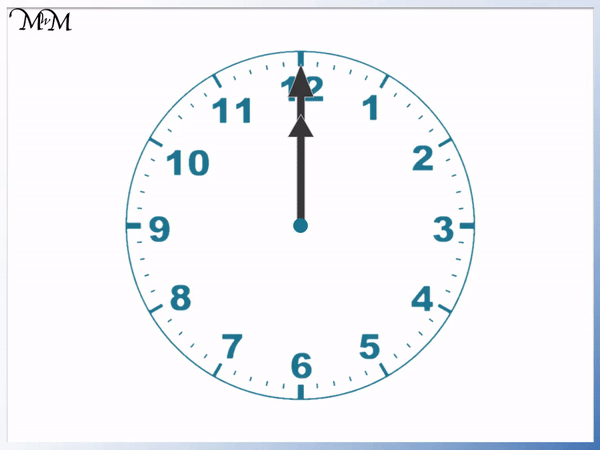We try to use minutes less than 30 to describe the time. If the minutes past the hour are between 0 and 30, then we use minutes past.

If the minutes past the hour are between 30 and 60, then we use minutes to the hour instead.

Basically, if the minute hand is before the 6, we use minutes past and if the minute hand is after the 6, we use minutes to the hour.We can count back from the hour in minutes to see the minutes to the next hour.Each mark is worth 1 minute. We can see that the number 11 is 5 minutes away from the next hour. Each extra number on the clock that we count is worth another 5 minutes.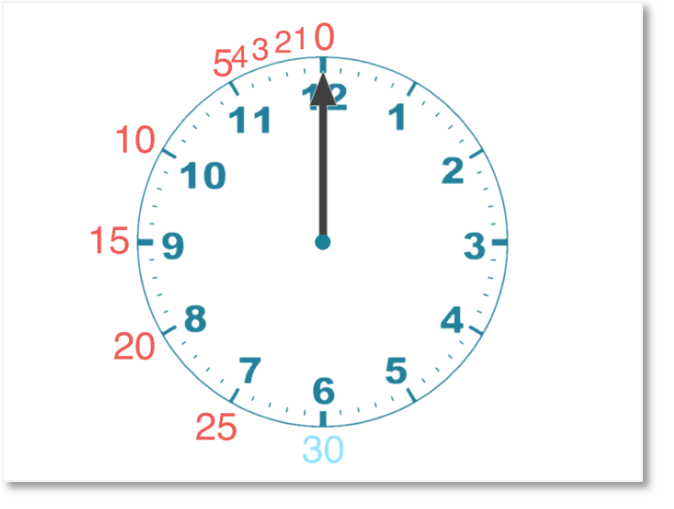Here is an example of reading minutes to the hour.

The minute hand is pointing at the 11. This is one number away from 12 at the top and so, this is one lot of 5 minutes to the next hour.

The minute hand pointing at 11 means 5 minutes to the next hour.We look at the shorter hour hand to see what the hour part of the time is.

The hour hand is between the 1 and the 2. It is very close to the 2 but not fully pointing at it yet.

In 5 minutes, it will be 2 o’clock.

The time is 5 minutes to 2.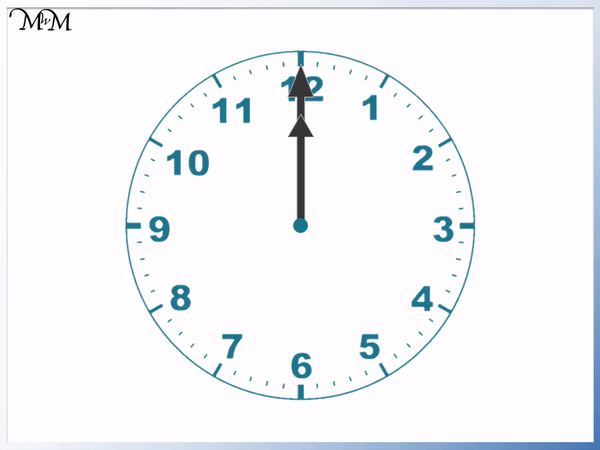Here is another example of telling the time to 5 minute intervals.

The minute hand is pointing at the 10.

The number 10 is two numbers away from the 12 at the top. This means we have two blocks of 5 minutes until the next hour.

We are 10 minutes to the next hour.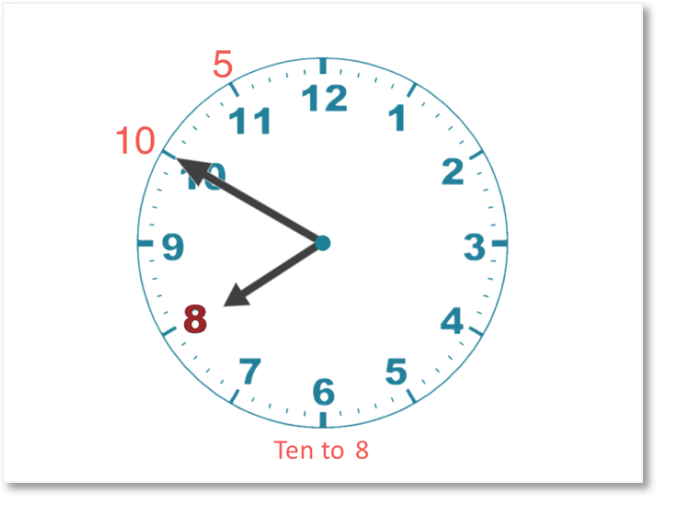The hour hand is between 7 and 8. It has already gone past 7 but not yet reached 8.

The next hour is 8 o’clock. This is in 10 minutes.

The time is 10 minutes to 8.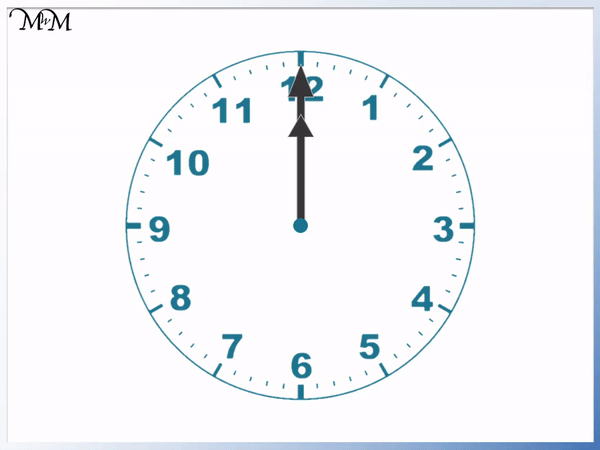Here is another example of telling the time in 5 minute intervals.

The minute hand is pointing at the 7. This number is five numbers away from the 12 at the top.

This means we have five lots of 5 minutes until the next hour.

The time is 25 minutes to the next hour.

In this example, it is easy to see that we are close to half past the hour, which is 30 minutes to the next hour. It is easier to take the 5 minutes off 30 to get 25 minutes to the hour.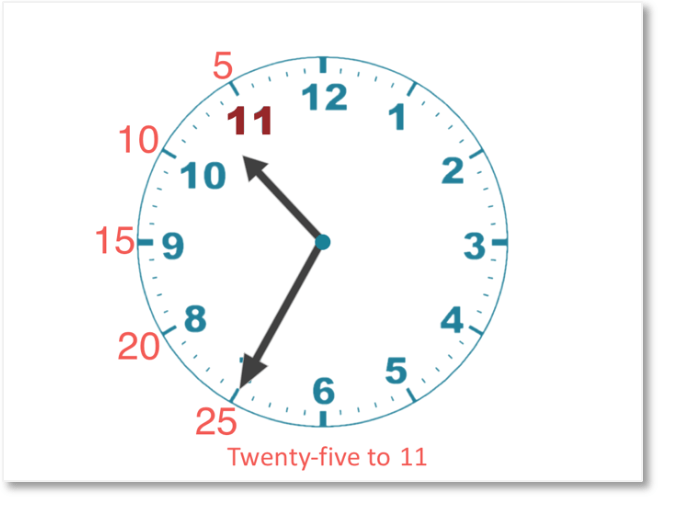The next hour is shown by the hour hand. It is in between 10 and 11.

This means that we have already had 10 o’clock and the next hour is 11 o’clock.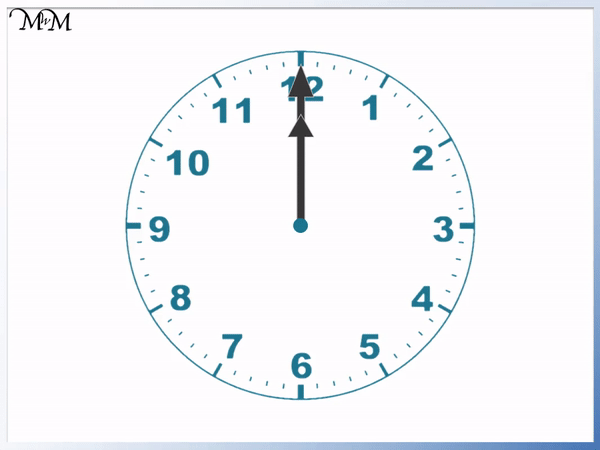The time is 25 minutes to 11.Now try our lesson on How to Read a Clock where we learn how to ready any time on a clock.